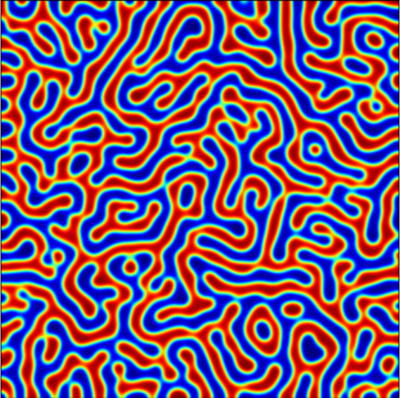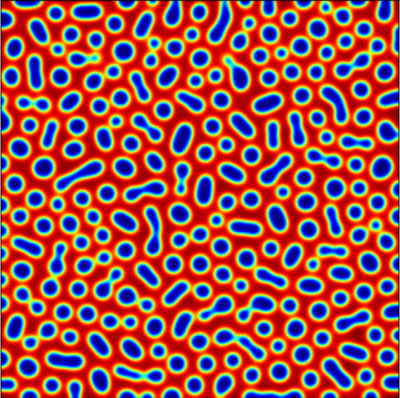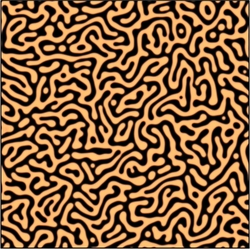Thomas Wanner
Department of Mathematical Sciences
George Mason University
4400 University Drive, MS 3F2
Fairfax, Virginia 22030, USA## Spindodal Decomposition in the Cahn-Hilliard Model

Part of my research has been focused on mathematical models for phase separation in binary alloys, in particular on the phenomenon of spinodal decomposition. Below you can see some movies of simulated phase separation in the Cahn-Hilliard model in two space dimensions, for a variety of values of the total mass $\mu$. To see the movies, just click on the images.

$\mu = 0.1$ $\mu = 0.2$ $\mu = 0.3$$\mu = 0.4$ $\mu = 0.5$ $\mu = 0.6$